Courses

# Conservation Laws and Symmetry - Lagrangian and Hamiltonian Equations, Classical Mechanics, CSIR-NET Physics Notes | EduRev

## Physics for IIT JAM, UGC - NET, CSIR NET

Created by: Akhilesh Thakur

## Physics : Conservation Laws and Symmetry - Lagrangian and Hamiltonian Equations, Classical Mechanics, CSIR-NET Physics Notes | EduRev

The document Conservation Laws and Symmetry - Lagrangian and Hamiltonian Equations, Classical Mechanics, CSIR-NET Physics Notes | EduRev is a part of the Physics Course Physics for IIT JAM, UGC - NET, CSIR NET.
All you need of Physics at this link: Physics

Symmetry in general

We take our first look at the relationship between the symmetries with a physical system and conservation laws in the special case of spatial symmetry.

In general, as far as classical mechanics is concerned, only continuous symmetries lead to conservation laws, although, in quantum mechanics, discrete symmetries do too.

symmetry associated with a system is defined to be a transformation of the coordinates of the system under which the Lagrangian, and hence the action, doesn't change.

In Euclidean space, the transformations are translations and rotations of the whole system.

Translational symmetry implies conservation of momentum

We have already seen a simple case of this in the last section, where we considered two particles on a line, each under the influence of a force due to a potential,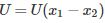, that only depended on the distance between the two particles.

If we apply a translation equally to both particles in the system,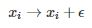the potential remains unchanged,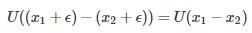The kinetic energy is also unchanged, since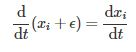Thus, both the potential and kinetic energy is invariant under translation, hence the Lagrangian and the action associated with the system are also translationally invariant. This is called translational symmetry.

As we have seen, specifying this potential implies that the sum of the internal forces is zero, hence the total momentum is conserved.

We shall consider a more general version of translational symmetry in the next lecture.

Partial symmetry

Consider a particle under the influence of gravity near the surface of the earth. Then, the potential is given by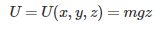In this case, a translation in either of the x,yx,y directions will not change the potential energy, but translation in the zz direction will. Thus, we only have two laws of momentum-conservation, rather than three.

Nb. The kinetic energy remains invariant under translations in all three directions.

Rotational symmetry implies implies conservation of angular momentum

It's clear that a given Lagrangian cannot depend on the coordinates used to describe a system of particles, so polar coordinates can be used.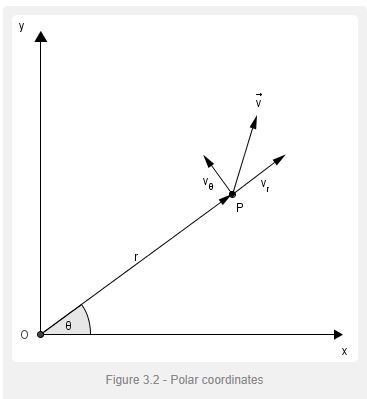Consider a particle in space subject to a central force - which means the force on the particle depends only on its distance to a given origin. Using polar cordinates, (r,θ), we can describe this using the potential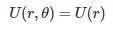Immediately we see that this potential is independent of θ, hence is invariant under changes in θ - that is, the potential is rotationally invariant.

We can write the velocity of the particle as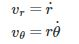and so the Lagrangian is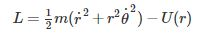We can calculate the Euler-Lagrange equations for (r,θ), starting with r,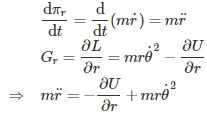Here we see a case where the generalised force Gr includes an extra term,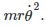This is an apparent force due to the angular velocity called the centrifugal pseudo-force.

Now the equation for θ,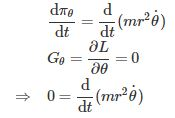From this it follows that the quantity,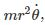is conserved. Of course, this is angular momentum, for which, (frustratingly) the symbol L is also used.

We can write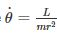and substitute into the equation above for r,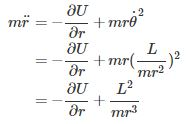and so we see that the centrifugal force is due to angular momentum. Note that it's proportional to the inverse cube of r, so will get very strong close to the origin.

159 docs

,

,

,

,

,

,

,

,

,

,

,

,

,

,

,

,

,

,

,

,

,

,

,

,

,

,

,

;